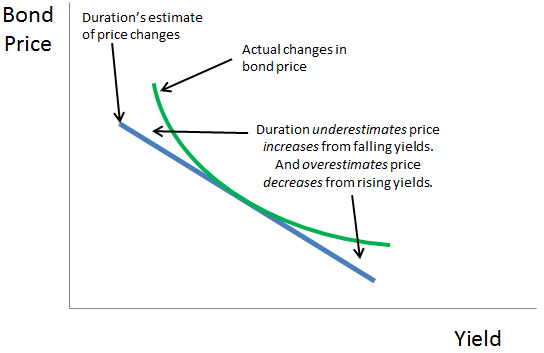Fixed-Income ETFs: Understanding Duration

"Bond prices go down when interest rates go up." That's the oft-repeated maxim in fixed-income investing, and mathematically, all else equal, it's true. But prices for some bonds fall more than others given the exact same change in interest rates. Understanding the basic drivers of interest-rate risk can help you identify which bonds make the most sense for you, and can help you identify which bonds are best to own—or best to avoid—if you think interest rates will increase.

In general, bond values decrease when interest rates increase, because investors can get a better deal in a rising-rate environment. Imagine a bond that's fairly priced and paying a 4 percent coupon. That coupon—another word for the regular interest payment—is typically a fixed cash amount, say, 4 percent of a \$100 par bond, or \$4. If interest rates suddenly increase, a newly issued bond that's very similar to the original bond might carry a 5 percent coupon, paying \$5 annually. Given the choice, who would wish to own the 4 percent coupon bond? Well, no one, if the price remained \$100. Instead, the market value or price of the 4 percent coupon bond would decrease to make it comparable to the yield of the 5 percent bond. If you own the 4 percent bond, you still receive your \$4 per year, but the bond's market value in your portfolio—the price you'd get if you sold it today—decreases.

But even "similar" bonds aren't identical, and different bonds will react very differently to the same movement in rates. Fortunately, the building blocks of interest-rate risk are fairly intuitive to grasp, starting with maturity. All else equal, a 10-year bond carries more interest-rate risk than a five-year bond, for example. This makes sense because your money is subject to the risk of rising interest rates for a longer period of time.

The statistic used to measure this time-weighted interest-rate risk is called "duration." A higher time-weighted average—a higher duration—means more interest-rate risk, and clearly it will take longer for you to get paid for a 10-year bond than for a five-year bond.

Understanding Duration

While duration can be calculated and expressed in different ways, at its core it conveys the interest-rate risk in a bond or a fixed-income portfolio. Whereas credit risk conveys the amount of risk related to the possibility of default, duration conveys the degree of risk associated with interest-rate movements. Duration is literally an estimate of the change in a bond's value in response to an overall change in interest rates.

For example, a duration of 4.3 means a bond's value will decrease 4.3 percent given a 1 percent increase in interest rates, and vice versa.

Why is duration so important? A change in the interest-rate environment can greatly affect the value of a bond or portfolio of bonds—such as an ETF. Duration provides a way to quantify this risk. This allows investors to directly compare interest-rate risk across bonds, bond portfolios and bond ETFs.

Few financial metrics are as seemingly simple and powerful as duration, but as usual, caveats apply.

First, duration is a forward-looking estimate, not a certainty. As we have stressed in this article, a bond's value goes up when interest rates go down. Although this is true, it doesn't follow a straight line—which is how duration estimates the relationship. The difference between duration's estimates of changes in bond prices and actual changes can be seen in the chart below.Second, duration calculations assume that the interest-rate environment moves in a particular way that isn't typical in real life. Specifically, duration assumes that interest rates change by 1 percent uniformly across all maturities. In other words, duration is calculated assuming that the entire yield curve shifts up or down by 1 percent—the five-year goes from 1 percent to 2 percent, the 10-year goes from 3 percent to 4 percent, and so on. Reality rarely works so precisely.

Still, duration provides a common framework for evaluating interest risk. In fact, duration is sometimes expressed in years. You can think of duration as the weighted average of all the cash flows from the bond over time; that is, all the coupons and the return of principal paid by the bond issuer to the investor. This way of thinking about duration provides an intuitive sense of when duration is likely to be higher or lower. In the example above, a longer maturity bond has higher weighted average cash flows (compared with an otherwise-identical shorter-dated bond), and therefore higher duration.

Taken a step further, we can imagine that the size of the coupon payments directly affects the weighted average of the cash flows for the bond too, and therefore its duration. A bond with larger coupon payments—where you get more cash back sooner—will have lower duration, while a bond with smaller coupon payments will have higher duration.

In real-world terms, that means a high-yield bond, which carries higher credit risk, could have lower interest-rate risk (compared with a similar investment-grade bond) because it makes higher coupon payments.

Combined with a basic understanding of credit risk, understanding a bond—or an ETF tracking a pool of bond duration—sets the stage for successful fixed-income investing.

Next: Fixed-Income ETFs: Introduction To Bank Loans

Other Articles Of Interest